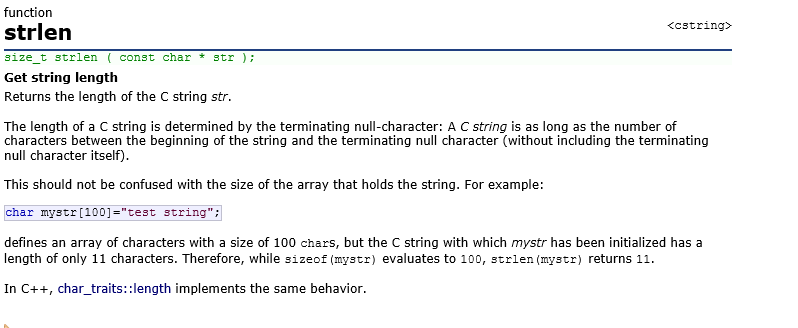# c语言数组遇上sizeof和strlen

2017-11-26 11:39 100 查看

## 数组的一些运算

```//一维数组
int a[] = {1,2,3,4};
printf("%d\n",sizeof(a));//16(表示数组大小)
printf("%d\n",sizeof(a+0));//4（a地址）
printf("%d\n",sizeof(*a));//4（对地址解引用，表示首元素）
printf("%d\n",sizeof(a+1));//4（aa的地址）
printf("%d\n",sizeof(a));//4（a元素）
printf("%d\n",sizeof(&a));//4（数组的地址）（还是地址就是4）
printf("%d\n",sizeof(&a+1));//4（跳过该数组的下一个数组的地址）（即4后面的地址）
printf("%d\n",sizeof(&a));//4（a的地址）
printf("%d\n",sizeof(&a+1)); //a的地址```

1，sizeof内部单独存放数组名，此时表示整个数组

2，&（数组名），此时表示整个数组

```//字符数组
char arr[] = {'a','b','c','d','e','f'};
printf("%d\n", sizeof(arr));//6(整个数组的大小)
printf("%d\n", sizeof(arr+0));//4（首元素的地址）
printf("%d\n", sizeof(*arr));//1（首元素字符）
printf("%d\n", sizeof(arr));//1（第二个元素字符）
printf("%d\n", sizeof(&arr));//4（数组的地址）
printf("%d\n", sizeof(&arr+1));//4（数组的地址，指向f后面）
printf("%d\n", sizeof(&arr+1));  //4（第二个元素的地址）

char arr[] = "abcdef";
printf("%d\n", sizeof(arr));//7(整个数组的大小)
printf("%d\n", sizeof(arr + 0));//4（首元素的地址）
printf("%d\n", sizeof(*arr));//1（首元素字符）
printf("%d\n", sizeof(arr));//1（第二个元素字符）
printf("%d\n", sizeof(&arr));//4（数组的地址）
printf("%d\n", sizeof(&arr + 1));//4（数组的地址，指向f后面）
printf("%d\n", sizeof(&arr + 1));//4（第二个元素的地址）

char arr[] = { 'a', 'b', 'c', 'd', 'e', 'f' };
printf("%d\n", strlen(arr));//随机值
printf("%d\n", strlen(arr + 0));//随机值
printf("%d\n", strlen(*arr));//ERR:strlen将97当作地址，不允许访问该空间
printf("%d\n", strlen(arr));//ERR:strlen将98当作地址，不允许访问该空间
printf("%d\n", strlen(&arr));//随机值（从a开始）
printf("%d\n", strlen(&arr + 1));//随机值（从f后开始数）
printf("%d\n", strlen(&arr + 1));//随机值（从b开始）

char arr[] = "abcdef";
printf("%d\n", strlen(arr));//6
printf("%d\n", strlen(arr + 0));//6(第一个元素开始数起)
printf("%d\n", strlen(*arr));//ERR:strlen将97当作地址，不允许访问该空间
printf("%d\n", strlen(arr));//ERR:strlen将98当作地址，不允许访问该空间
printf("%d\n", strlen(&arr));//6
printf("%d\n", strlen(&arr + 1));//随机值（跳过整个数组）
printf("%d\n", strlen(&arr + 1));//5（从b开始）

char *p = "abcdef";
printf("%d\n", sizeof(p));//4
bcaa
（指针变量）
printf("%d\n", sizeof(p + 1));//4（地址）
printf("%d\n", sizeof(*p));//1（访问一个字符）
printf("%d\n", sizeof(p));//1（第一个元素字符）
printf("%d\n", sizeof(&p));//4（指针的地址）
printf("%d\n", sizeof(&p + 1));//4（p地址的下一个地址）
printf("%d\n", sizeof(&p + 1));//4（b的地址）

printf("%d\n", strlen(p));//6
printf("%d\n", strlen(p + 1));//5
printf("%d\n", strlen(*p));//ERR：strlen(97)不允许访问
printf("%d\n", strlen(p));//ERR
printf("%d\n", strlen(&p));//随机值（p的地址向后找）
printf("%d\n", strlen(&p + 1));//随机值（跳过p的地址向后找）
printf("%d\n", strlen(&p + 1));//5（b的地址向后找）```

sizeof:运算符。功能：获得保证能容纳实现所建立的最大对象的字节大小。

sizeof后面如果是类型必须加（），变量名可以不加。（建议：最好都加）

strlen：函数。